# KR101035184B1 - Semiconductor test apparatus - Google Patents

## Info

Publication number
KR101035184B1
KR101035184B1 KR1020047020030A KR20047020030A KR101035184B1 KR 101035184 B1 KR101035184 B1 KR 101035184B1 KR 1020047020030 A KR1020047020030 A KR 1020047020030A KR 20047020030 A KR20047020030 A KR 20047020030A KR 101035184 B1 KR101035184 B1 KR 101035184B1
Authority
KR
South Korea
Prior art keywords
signal
data
information
cross point
timing
Prior art date
Application number
KR1020047020030A
Other languages
Korean (ko)
Other versions
KR20050007601A (en
Inventor
도시유끼 오까야스
마사또시 오하시
Original Assignee
가부시키가이샤 어드밴티스트
Priority date (The priority date is an assumption and is not a legal conclusion. Google has not performed a legal analysis and makes no representation as to the accuracy of the date listed.)
Filing date
Publication date
Priority to JPJP-P-2002-00168304 priority Critical
Priority to JP2002168304 priority
Application filed by 가부시키가이샤 어드밴티스트 filed Critical 가부시키가이샤 어드밴티스트
Priority to PCT/JP2003/007315 priority patent/WO2003104826A1/en
Publication of KR20050007601A publication Critical patent/KR20050007601A/en
Application granted granted Critical
Publication of KR101035184B1 publication Critical patent/KR101035184B1/en

• 239000004065 semiconductors Substances 0.000 title claims abstract description 51
• 238000005070 sampling Methods 0.000 claims description 22
• 238000006243 chemical reactions Methods 0.000 claims description 13
• 230000000875 corresponding Effects 0.000 claims description 11
• 238000005259 measurements Methods 0.000 claims description 2
• 230000000694 effects Effects 0.000 description 3
• 238000004519 manufacturing process Methods 0.000 description 1

## Images

•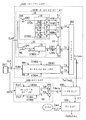•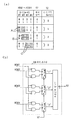•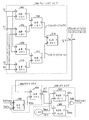•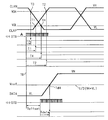•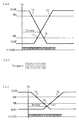•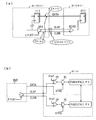•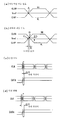•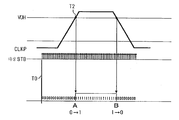•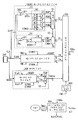•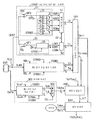•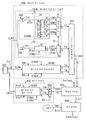•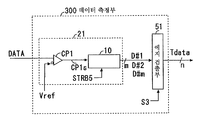•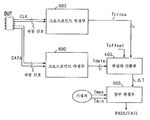## Classifications

• GPHYSICS
• G01MEASURING; TESTING
• G01RMEASURING ELECTRIC VARIABLES; MEASURING MAGNETIC VARIABLES
• G01R31/00Arrangements for testing electric properties; Arrangements for locating electric faults; Arrangements for electrical testing characterised by what is being tested not provided for elsewhere
• G01R31/28Testing of electronic circuits, e.g. by signal tracer
• G01R31/317Testing of digital circuits
• G01R31/3181Functional testing
• G01R31/319Tester hardware, i.e. output processing circuit
• G01R31/3193Tester hardware, i.e. output processing circuit with comparison between actual response and known fault free response
• G01R31/31937Timing aspects, e.g. measuring propagation delay
• GPHYSICS
• G01MEASURING; TESTING
• G01RMEASURING ELECTRIC VARIABLES; MEASURING MAGNETIC VARIABLES
• G01R31/00Arrangements for testing electric properties; Arrangements for locating electric faults; Arrangements for electrical testing characterised by what is being tested not provided for elsewhere
• G01R31/28Testing of electronic circuits, e.g. by signal tracer
• G01R31/317Testing of digital circuits
• G01R31/3181Functional testing
• G01R31/319Tester hardware, i.e. output processing circuit
• G01R31/31903Tester hardware, i.e. output processing circuit tester configuration
• G01R31/31908Tester set-up, e.g. configuring the tester to the device under test [DUT], down loading test patterns
• G01R31/3191Calibration
• GPHYSICS
• G01MEASURING; TESTING
• G01RMEASURING ELECTRIC VARIABLES; MEASURING MAGNETIC VARIABLES
• G01R31/00Arrangements for testing electric properties; Arrangements for locating electric faults; Arrangements for electrical testing characterised by what is being tested not provided for elsewhere
• G01R31/28Testing of electronic circuits, e.g. by signal tracer
• G01R31/317Testing of digital circuits
• G01R31/3181Functional testing
• G01R31/319Tester hardware, i.e. output processing circuit
• G01R31/31917Stimuli generation or application of test patterns to the device under test [DUT]
• G01R31/31922Timing generation or clock distribution

## Abstract

The cross point of the differential clock signal CLK output from the DUT and the timing of the both signals of the data signal DATA are measured and the relative phase difference between the both signals is determined. Differential signal timing measurement means for outputting cross point information Tcross obtained by measuring the timing of a cross point of one differential output signal outputted from the DUT, and a comparator for comparing the logic of the other non-differential output signal output from the DUT A non-differential signal timing measurement means for outputting data change point information Tdata obtained by measuring the transition timing and a phase difference DELTA T obtained by obtaining a relative phase difference between the cross point information Tcross obtained by simultaneously measuring both output signals and the data change point information Tdata And positive and negative determination means for determining the relative phase relationship of the threshold value DUT on the basis of the predetermined threshold value for performing the positive / negative determination in response to the phase difference? T.
Semiconductor test, differential clock signal, crosspoint, phase difference calculation, signal timing measurement

## Description

SEMICONDUCTOR TEST APPARATUS

The present invention relates to a semiconductor test apparatus for testing a device under test (DUT) that outputs a differential clock signal. In particular, the present invention relates to a semiconductor test apparatus for testing a relative phase difference between a cross point of one differential clock signal CLK output from a DUT and the other data signal DATA output from the DUT.

The background art relating to the present invention is disclosed. In Japanese Patent Application No. 2000-178917 (Semiconductor Device Test Method and Semiconductor Device Test Apparatus), a test method for testing a semiconductor device that outputs a reference clock DQS used for transferring data in synchronism with data reading in a short period of time is solved .

In Japanese Patent Application 2000-9113 (semiconductor device testing method and semiconductor device testing apparatus), a test method for testing a semiconductor device that outputs a reference clock DQS used for transferring data in synchronization with data reading in a short time with high accuracy .

Japanese Patent Application No. 2000-204757 (Semiconductor Device Test Method and Semiconductor Device Test Apparatus) discloses a semiconductor device that outputs a reference clock in synchronization with a readout output of data and provides the reference clock to the transfer of data, And a test method for judging a failure according to a phase difference between a clock and data is solved.

However, in these background arts, since the cross point of the differential clock signal CLK can not be specified, it is not possible to accurately determine the relative phase difference between the other signals.

Next, problems according to the present invention will be described.

6A is a schematic circuit connection in a case where high-speed data transfer is performed in synchronism with a differential clock output as a balanced signal between two devices on a circuit board or the like, and differential transmission such as ECL or LVDS .

The differential clock signal CLK (positive clock signal CLKP, negative clock signal CLKN) is output from the differential driver DR1 of the first device and supplied to the differential receiver RCV2 of the second device through the transmission line. One or a plurality of data signals DATA synchronized with the clock from the flip-flop FF1 of the first device are supplied to the input terminal of the flip-flop FF2 of the second device and are retimed to the clock by the differential receiver RCV2 . Incidentally, the differential clock signal has a variation in the output amplitude due to the manufacturing deviation of the IC, a phase error between the differential signals accompanying the circuit configuration, and the like. In some cases, the differential clock signal or data DATA may include some jitter components or generate common-mode noise.

Taking these into consideration, it is demanded that the first device that becomes the DUT (device under test) outputs the output relationship between clock and data in a prescribed phase relation. In the semiconductor testing apparatus, it is required that the difference between the differential clock signal and the data DATA can be judged by measuring whether or not the differential clock signal is output in a prescribed phase relationship. In addition, since the differential driver DR1 can turn off the output to suppress the high impedance state, there is a need to test the differential driver DR1.

6B shows a comparator (CP) used in a single-ended (unbalanced) form to receive the respective signals of the positive clock signal CLKP and the sub clock signal CLKN, which are differential clock signals output from the DUT The semiconductor testing apparatus according to claim 1, Here, since the semiconductor testing apparatus needs to measure each signal of the differential clock signal output from the DUT, the semiconductor testing apparatus is configured to receive the signal separately from the two-channel single-ended comparator CP. This is because, for example, the DUT differential clock signal has a test item in a high impedance state (Hi-Z mode) and needs to be testable.

6, the first comparator CP receives one positive clock signal CLKP, converts the positive clock signal CLKP into a logic signal at a predetermined threshold level Vref, receives the positive clock signal CLKP, and outputs the logic signal to the timing comparator TC so that the signal held by the strobe signal STRB Quot ;. &lt; / RTI &gt;

The second comparator CP also receives the other sub clock signal CLKN and converts it into a logic signal at a predetermined threshold level Vref and then receives the clock signal CLKN and supplies it to the timing comparator TC to generate a logic signal based on the signal held by the strobe signal STRB at a desired timing. An affirmative decision on the single-ended signal of the individual input is made.

Here, in the case of the ideal differential signal shown in Fig. 7A, the cross point (point A in Fig. 7) of the clock signal is shifted from the voltage of the intermediate voltage threshold level Vref by half of the amplitude to the logic signal Conversion.

However, as shown in the example of the actual differential signal of Fig. 7B, when the signal is converted from the threshold level Vref to the logic signal, the cross point (Fig. 7B) Point &quot; C &quot;). As a result, there is a difficulty in generating a timing error (E difference in Fig. 7) between both cross points, and the accuracy of the timing measurement deteriorates. Particularly, when the clock frequency becomes several hundred MHz or more, the influence of the measurement accuracy becomes large. Since the semiconductor testing apparatus is a measuring apparatus requiring a high-precision timing measurement, this is a practical difficulty.

7C shows a case where both signals are changed in the same phase in accordance with a jitter factor such as inter-signal interference noise / power supply noise in the simultaneous measurement of both the differential clock signal CLK and the data signal DATA. In this case, the relative phase difference f between the two signals is small. It is necessary to measure the instantaneous phase difference DELTA f1 to determine whether or not it is within the normal phase range.

In contrast, FIG. 7 (d) shows a case where both signals are reversed in phase with the jitter factor in the simultaneous measurement of both the differential clock signal CLK and the data signal DATA. In this case, the relative phase difference f of the instant between the two signals becomes large. It is necessary to measure the instantaneous phase difference? F2 accompanying the above-described jitter factor, and to judge whether or not it is within the normal phase range. Thus, there is a need to measure the relative phase difference between the two signals at the same time and to judge whether the two signals are correct.

As described above, the two-channel single-ended comparator CP is used to specify the position of the cross point of the differential clock signal CLK. This is because the cross point of the positive clock signal CLKP and the negative clock signal CLKN As a result of the movement, it can not be precisely specified.

In order to accurately evaluate the phase between the differential clock signal CLK and the data signal DATA, both signals are sampled at the same time, the cross point of the differential clock signal CLK is specified, It is required to evaluate the phase between the data signal DATA.

However, in the prior art, the relative phase difference between the cross point of the differential clock signal CLK and both signals of the data signal DATA can not be accurately judged and can not be determined. Since the semiconductor testing apparatus is a measuring apparatus requiring timing measurement with high accuracy, this is not preferable and is a practical difficulty.

Therefore, a problem to be solved by the present invention is to measure and specify the timing of a cross point of one differential clock signal output from the DUT by applying a two-channel single-ended comparator CP, The present invention provides a semiconductor testing apparatus which can measure the timing of DATA and determine the relative phase difference between the two signals from this to realize a good device judgment.

It is another object of the present invention to provide a semiconductor testing apparatus which can accurately measure the cross point of a differential clock signal output from a DUT by applying a two-channel single-ended comparator CP.

Another object of the present invention is to provide a semiconductor testing apparatus capable of specifying a relative phase difference between a differential signal output from a DUT and another differential signal outputted from a DUT or a differential signal. It is another object of the present invention to provide a semiconductor testing apparatus capable of measuring a relative jitter amount between a differential signal output from a DUT and another signal output from a DUT.

A first solution means of the present invention is disclosed. Here, FIG. 4 and FIG. 1 show the solution means according to the invention.

In order to solve the above problem, a differential signal timing measuring means (for example, a cross point measuring means) for outputting cross point information Tcross obtained by measuring a timing of a cross point of one differential output signal outputted from a DUT (600)),

Differential signal timing measuring means (for example, a data measuring unit (not shown) for outputting data change point information Tdata obtained by measuring the transition timing at which the logic of the other non-differential (single-ended) 300)

(For example, a phase difference calculating section 400) for obtaining a relative phase difference between the cross point information Tcross obtained by simultaneously measuring both output signals and the data change point information Tdata,

A positive / negative determination means (for example, a positive / negative determination means (for example, a positive / negative determination means) for determining the relative phase relationship of the DUT based on a predetermined upper limit threshold value and a lower threshold value or one threshold value for receiving the phase difference? 500), and is provided with the semiconductor test apparatus.

According to the present invention, the timing of the cross point of one differential signal outputted from the DUT is measured and specified by applying the two-channel single-ended comparator CP, and the timing of the other data signal DATA output from the DUT is measured And by obtaining the relative phase difference between the two signals from this, it is possible to realize a semiconductor testing apparatus capable of realizing good device fairness determination.

Next, a second solution means is disclosed. Here, Fig. 13 shows the solution means according to the invention.

A first differential signal timing measurement means (for example, a cross point measurement section) for outputting first cross point information Tcross obtained by measuring the timing of a cross point of one of the first differential output signals outputted from the DUT, (600)),

(For example, the cross point measuring unit 600) for outputting the second cross point information Tcross obtained by measuring the timing of the cross point of the other second differential output signal outputted from the DUT, And,

A phase difference calculating unit (for example, a phase difference calculating unit (for example, a phase difference calculating unit) for calculating a phase difference? T obtained by obtaining a relative phase difference between the first cross point information Tcross and the second cross point information Tcross obtained by simultaneously measuring both differential output signals 400)

A positive / negative determination means (for example, a positive / negative determination means (for example, a positive / negative determination means) for determining the positive and negative sides of the DUT based on a predetermined upper limit threshold value and a lower limit threshold value or one threshold value corresponding to the DUT, 500), and has a semiconductor testing apparatus as described above.

Thus, relative phase difference can be determined by specifying the relative phase difference of the two systems of differential signals.

Next, a third solution means is disclosed. 4, Fig. 5 and Fig. 1 show the solution according to the invention.

In order to solve the above problem, it is desirable to measure the relative phase difference between the data signal DATA output from the DUT and the other data signal DATA with good precision, based on the timing of the cross point of one differential output signal output from the DUT In the required semiconductor testing apparatus,

A transition waveform of one signal in the differential output signal is converted into a logic signal at a predetermined threshold level VOH and VOL at which two points are measured before and after the cross point and then sampled and measured based on a polyphase strobe signal at a known timing (For example, first transition time information collecting means 100 # 1) for outputting two pieces of timing information converted into code data,

A transition waveform of the other signal in the differential output signal is converted into a logic signal at a predetermined threshold level VOH and VOL at which two points are measured before and after the cross point, (For example, second transition time information collecting means 100 # 2) for outputting two pieces of timing information converted into code data,

Between a first straight line passing between two timing information obtained from a transition waveform of one signal in the differential output signal and a second straight line passing between two timing information obtained from a transition waveform of the other signal in the differential output signal (For example, a cross point calculating section 200) for specifying, as a cross point information Tcross, a position at which a straight line intersects on a second straight line passing therethrough,

The other data signal DATA output from the DUT is received and converted into a logic signal at a predetermined threshold level Vref and then sampled and measured based on a polyphase strobe signal at a known timing. (For example, a data measuring unit 300) for outputting data change point information Tdata converted into code data representing one of the timing information,

(For example, a phase difference calculating section 400) for obtaining and outputting a relative phase difference? T between the cross point information Tcross and the data change point information Tdata,

(For example, the positive / negative determination section 500) that receives the phase difference? T and determines whether or not the phase difference is within the specification of the phase difference with respect to the DUT varieties. There is a testing device.

Next, a fourth solution means is disclosed. Here, FIG. 4 and FIG. 1 show the solution means according to the invention.

In order to achieve the above object, a device under test is a device for outputting a differential output signal (for example, a positive clock signal and a sub clock signal) and at least one data signal DATA synchronized with the differential output signal, (Unbalanced) by an analog comparator, and has a structure in which a signal of a data signal DATA (DATA) when the timing of a cross point at which both positive signals of the differential output signal outputted from the DUT cross In a semiconductor testing apparatus in which it is required to measure the relative phase difference of the semiconductor wafer with good precision,

The signal is converted into a logic signal at a two-point threshold level of a predetermined low level and a high level for generating a cross point with respect to a transition waveform of a signal of the differential output signal. Thereafter, based on the polyphase strobe signal of known timing, (For example, first transition time information collecting means 100 # 1) for outputting first timing information T1 and second timing information T2 converted into code data,

A logic level conversion circuit for converting a logic level of a transition waveform of the other signal of the differential output signal into a logic level at a predetermined high level and a low level at two threshold levels for generating a cross point, (For example, second transition time information collecting means 100 # 2) for outputting third timing information T3 and fourth timing information T4 obtained by sampling measurement and converted into code data,

Based on the first timing information T1 and the second timing information T2 obtained from one of the transition waveforms, the first line passing through the transition waveform and the third timing information T3 and the fourth timing information T4 obtained from the other transition waveform And a cross point calculating means (for example, a cross point calculating section 200) for finding, as cross point information Tcross, a position at which the straight line of the second straight line passing through the transition waveform crosses,

The data signal DATA output from the DUT is converted into a logic signal at a predetermined threshold level Vref and then subjected to sampling measurement based on a polyphase strobe signal at a known timing to generate code data indicating the timing of the rise or fall of the data signal DATA And a data transition time information collection means (for example, a data measurement unit 300) for outputting the converted data change point information Tdata,

(For example, a phase difference calculating section 400) for obtaining and outputting a relative phase difference? T between the cross point information Tcross and the data change point information Tdata,

(For example, an affirmative judgment section 500 (for example, a negative judgment section) for judging whether or not the obtained phase difference? T is within the specification of the phase difference for the DUT varieties (for example, the maximum phase difference Tmax and the minimum phase difference Tmin) ), And has a semiconductor testing apparatus as described above.

Next, a fifth solution means is disclosed. Here, Figure 5 shows the solution according to the invention.

In order to solve the above problems, there is provided a semiconductor testing apparatus which is required to measure a timing of a cross point of a differential output signal outputted from a device under test with good precision,

A transition waveform of one signal in the differential output signal is converted into a logic signal at a predetermined threshold level VOH and VOL at which two points are measured before and after the cross point and then sampled and measured based on a polyphase strobe signal at a known timing (For example, first transition time information collecting means 100 # 1) for outputting two pieces of timing information converted into code data,

A transition waveform of the other signal in the differential output signal is converted into a logic signal at a predetermined threshold level VOH and VOL at which two points are measured before and after the cross point, (For example, second transition time information collecting means 100 # 2) for outputting two pieces of timing information converted into code data,

Between a first straight line passing between two timing information obtained from a transition waveform of one signal in the differential output signal and a second straight line passing between two timing information obtained from a transition waveform of the other signal in the differential output signal And a cross point calculating means (for example, a cross point calculating section 200) for specifying, as the cross point information Tcross, a position where the straight lines intersect on the second straight line passing through the intersection, As shown in FIG.

Thus, the cross point of the differential signal can be accurately specified.

Next, a sixth solution means is disclosed. 1 shows a solution according to the invention.

One mode of the transition information measuring means (for example, the first transition time information collecting means 100 # 1 and the second transition time information collecting means 100 # 2) A second analog comparator CP2 for generating the second timing information T2 and a second polyphase strobe means 10 for generating the second timing information T2, each of which has a first analog comparator CP1, a first polyphase strobe means 10 and a first edge detector 52, And a second edge detecting unit 51,

The first analog comparator CP1 receives a signal output from the DUT and supplies a logic signal converted from a predetermined low level VOL to a logic signal to the first polyphase strobe means 10,

The first polyphase strobe means 10 receives a logic signal from the first analog comparator CP1 to generate a plurality of m polyphase strobe signals provided with micro-phase difference therein and generates a logic signal by the generated polyphase strobe signals (Here, i = 1 to m) of sampled plural m-bit low-side hold signals LD # i,

The first edge detection unit 52 receives the low-side hold signal LD # i of a plurality of m bits, and generates a first edge detection signal LD # i based on the edge selection signal S2 for selecting the rising or falling edge direction. 1 timing information T1,

The second analog comparator CP2 receives the signal output from the DUT and supplies a logic signal obtained by converting the signal from the predetermined high level VOH to the logic signal to the second polyphase strobe means 10,

The second polyphase strobe means 10 receives the logic signal from the second analog comparator CP2 and internally generates a plurality of m polyphase strobe signals to which micro-phase difference is given, and generates a logic signal by the generated polyphase strobe signals And outputs the sampled high-side hold signal HD # i of a plurality of m bits,

The second edge detector 51 receives the high-side hold signal HD # i of a plurality of m bits and converts the m-bit input to the n-bit output based on the edge selection signal S2 for selecting the rising or falling edge direction. 2 timing information T2. The above-mentioned semiconductor testing apparatus is characterized in that it is a data encoder for outputting two timing information T2.

Next, a seventh solution means is disclosed. 1 shows a solution according to the invention.

One aspect of the above-described data transition time information collecting means (for example, the data measuring unit 300) includes an analog comparator for generating the timing information T1, a polyphase strobing unit 10, a first edge detecting unit and a second edge detecting unit A multiplexer 350,

The analog comparator receives a data signal DATA of a non-differential signal output from the DUT and supplies a logic signal obtained by converting the data signal DATA to a logic signal at a predetermined threshold level Vref to the polyphase strobe means 10,

The multiphase strobe means 10 receives a logic signal from the analog comparator and generates a plurality of m multiphase strobe signals provided with micro-phase difference therein. The multiphase strobing means 10 generates a plurality of m And outputs a hold signal D # i of the bit (here, i = 1 to m)

The first edge detection unit receives the hold signal D # i of a plurality of m bits and generates timing information Tdh of one rising side which is obtained by encoding conversion of the m-bit input into n-bit output based on the edge selection signal S2 for selecting the rising edge direction And outputting the encoded data,

The second edge detection unit receives the hold signal D # i of a plurality of m bits and outputs the timing information Tdl of the other side on which the m-bit input is encoded into the n-bit output based on the edge selection signal S2 for selecting the falling edge direction And outputs the data,

The multiplexer 350 selects either one of the rising edge timing information Tdh and the falling edge timing information Tdl on the basis of the data edge selection signal S3 for selecting the data edge and outputs it as the data change point information Tdata The semiconductor test apparatus described above.

Next, an eighth solution means is disclosed. Here, Fig. 12 shows the solution means according to the invention.

One aspect of the above-described data transition time information collection means (for example, the data measurement unit 300) includes an analog comparator for generating the timing information T1, a polyphase strobe unit 10 and an edge detection unit,

The analog comparator receives a data signal DATA of a non-differential signal output from the DUT and supplies a logic signal obtained by converting the data signal DATA to a logic signal at a predetermined threshold level Vref to the polyphase strobe means 10,

The multiphase strobe means 10 receives a logic signal from the analog comparator and generates a plurality of m multiphase strobe signals provided with micro-phase difference therein. The multiphase strobing means 10 generates a plurality of m Bit hold signal D # i,

The edge detection unit receives the hold signal D # i of a plurality of m bits and generates a data change point which is obtained by encoding the m-bit input into the n-bit output based on the data edge selection signal S3 for selecting the data edge in the rising edge direction or the falling edge direction. And a data encoder for outputting information Tdata.

Next, a ninth solution means is disclosed. 3 and 5 show the solution means according to the invention.

One aspect of the above-mentioned cross point calculating means is such that the first timing information obtained by the first transition information measuring means is T1, the second timing information is T2, the third timing information obtained by the second transition information measuring means is T3 And the fourth timing information is T4,

Tcross = {(T2.times.T4) - (T1.times.T3)} / {(T2-T1) + (T4-T3)}

(For example, a cross point calculating section 200) for generating and outputting cross point information Tcross subjected to the arithmetic processing of the cross point information Tcross.

Next, a tenth solution means is disclosed. Here, Fig. 11 shows the solution means according to the invention.

One aspect of the above-mentioned cross point calculating means includes the cross point conversion memory 250 for data conversion,

The first timing information obtained by the first transition information measuring means is T1, the second timing information is T2, the third timing information obtained by the second transition information measuring means is T3, and the fourth timing information is T4 When you do,

The cross point conversion memory 250 stores the cross point information Tcross corresponding to the above-described operation processing in advance in the memory, supplies data of the timing information T1, T2, T3, and T4 to the address input terminal, And outputs the read data as cross-point information Tcross.

Next, an eleventh solution means is disclosed. Here, Fig. 3 shows a solution according to the invention.

As one aspect of the above-described phase difference calculating means, the cross point information Tcross from the cross point calculating means and the data change point information Tdata from the data transition time information collecting means (for example, the data measuring unit 300) Outputting a relative phase difference DELTA T that calculates the difference of data or outputting a phase difference DELTA T as a result of further adding a predetermined offset amount (offset time Toffset) to the phase difference DELTA T (for example, )). &Lt; / RTI &gt;

Next, a twelfth solution means is disclosed. Here, Fig. 3 shows a solution according to the invention.

As an aspect of the above-described good and good determining means, the relative phase difference DELTA T is received from the phase difference calculating means, and the positive / negative determination of the DUT is made based on whether the predetermined maximum phase difference Tmax is within the allowable range of the minimum phase difference Tmin (For example, the positive / negative determination section 500).

Next, a thirteenth solution means is disclosed. Here, Fig. 10 shows the solution means according to the invention.

The above-mentioned cross-point calculating means and the above-mentioned correcting means are additionally provided,

The positive decision control means is provided with a first timing of four points output from the transition information measuring means (for example, the first transition time information collecting means 100 # 1 and the second transition time information collecting means 100 # 2) When the data value of at least one of the information T1, the second timing information T2, the third timing information T3 and the fourth timing information T4 is '0', it is determined that the normal cross point is not measured and the data error signal Derr is cross- And the means for judging whether or not the data error signal Derr has been received is provided from the calculating means.

Next, a fourteenth solution means is disclosed. Here, Fig. 9 shows the solution means according to the invention.

In order to solve the above problems, there is a semiconductor test in which it is required to measure the relative phase difference between the differential output signal output from the DUT and the other data signal DATA output from the DUT with good precision, based on the timing of the cross point of the differential output signal outputted from the DUT In the apparatus,

The first timing information T1 and the second timing information T2, which have been converted into the code data by the sampling measurement based on the polyphase strobe signal of known timing and converted into the code data, are converted into the logic signals at the two threshold levels of the predetermined low level and high level, (For example, the first transition time information collecting means 100 # 1) for outputting the first transition time information,

A logic level conversion circuit for converting a logic level of a transition waveform of the other signal of the differential output signal into a logic level at a predetermined high level and a low level at two threshold levels for generating a cross point, (For example, second transition time information collecting means 100 # 2) for outputting third timing information T3 and fourth timing information T4 obtained by sampling measurement and converted into code data,

The data signal DATA output from the DUT is converted into a logic signal at a predetermined threshold level Vref and then subjected to sampling measurement based on a polyphase strobe signal at a known timing to generate code data indicating the timing of the rise or fall of the data signal DATA And a data transition time information collection means (for example, a data measurement unit 300) for outputting the converted data change point information Tdata,

The timing information of two points measured by the first transition information measuring means, the timing information of two points measured by the second transition information measuring means, and the timing information of one point measured by the data transition time information collecting means, (For example, edge data storage memory 700) having a predetermined storage capacity for storing the measured and stored data,

The data content of the edge data storage means is read and the relative phase difference? T between the cross point information Tcross and the data change point information Tdata calculated by calculating the cross point in software is calculated and calculated, (For example, a cross point calculation / phase difference calculation / phase difference calculation processing) for determining whether or not the obtained phase difference? T of the plurality of points is within the standard of the phase difference for the DUT type, And a positive / negative determination processing unit (650)), and has the above-described configuration.

Next, a fifteenth solution means is disclosed. Here, Fig. 9 shows the solution means according to the invention.

In the semiconductor testing apparatus described above, a plurality of points of phase difference DELTA T corresponding to the number of measurements obtained by the cross point calculation and positive / negative determination processing means are received, and the amount of fluctuation of the phase difference DELTA T at a plurality of points is obtained. The semiconductor testing apparatus further includes a function of adding a function for testing the semiconductor device.

Thus, the relative amount of jitter between both signals can be measured.

Further, the present invention may be applied to other constituent means that are practically usable by suitably combining the respective element means in the solution means as desired. The numerals given to the respective elements correspond to the numerals disclosed in the embodiments of the present invention and the like, but the present invention is not limited thereto, and constituent means using other practically usable equivalents may be used.

FIG. 1 is a block diagram showing a configuration of a main part of a semiconductor testing apparatus in a case where a differential clock signal outputted from a DUT and a single-ended data signal DATA are received and a relative phase difference between the differential clock signal and a differential signal is obtained.

2 is an example of encoding and an example of a circuit configuration of the edge detecting unit when m = 4 bits.

3 is a specific internal configuration example of the cross point calculating unit 200. As shown in FIG.

Fig. 4 is a simplified timing chart showing the timing information T1 to T4, Tdh when the strobe signals STRB1 to STRB4 occur at the same reference timing TO.

5 is an explanatory diagram of calculation of cross-point information Tcross.

6 shows a schematic circuit connection in case of performing high-speed data transfer in synchronism with a differential clock between two devices on a circuit board or the like, a positive clock signal CLKP as a differential clock signal output from the DUT, a negative clock signal CLKN End comparator CP in order to receive the respective signals of the comparator CP.

Fig. 7 is a diagram for explaining the in-phase variation and the reverse phase variation accompanying the jitter factor in the cross point in the case of the ideal differential signal, the cross point in the actual differential signal example, and both signals of the differential clock signal CLK and the data signal DATA .

8 is a timing chart showing an example of a case where the pulse width is narrow.

FIG. 9 shows another example of the main part block configuration of the semiconductor testing device in the case where the differential clock signal outputted from the DUT and the data signal DATA of the single end are received and the relative phase difference between them is obtained to perform the positive / negative judgment.

10 is another example of the configuration of the main part of the semiconductor testing apparatus in the case where the differential clock signal outputted from the DUT and the data signal DATA of the single end are received and the relative phase difference between the differential clock signal and the differential signal is obtained.

11 is another example of the configuration of a substantial block of the semiconductor testing apparatus in the case where the differential clock signal output from the DUT and the single-ended data signal DATA are received and the relative phase difference between them is obtained to perform the positive / negative determination.

FIG. 12 shows another configuration example of the data measuring unit 300. FIG.

Fig. 13 shows another configuration example in which the relative phase difference of the differential signals of the two systems is obtained to perform the positive / negative determination.

BEST MODE FOR CARRYING OUT THE INVENTION Hereinafter, an embodiment of the present invention to which the present invention is applied will be described with reference to the drawings. Furthermore, the claims are not intended to limit the scope of the claims according to the following description of the embodiments, and the elements and connection relationships described in the embodiments can not necessarily be essential to the solution. In addition, the form / form of the element or the connection relationship described in the embodiment is not limited to the form / form content as an example.

The present invention will be described below with reference to Figs.

FIG. 1 shows an example of the configuration of a main part of a semiconductor testing apparatus that receives a differential clock signal output from a DUT and a data signal DATA of a single end to obtain a relative phase difference between the two and determine whether the semiconductor test apparatus is correct. Further, the entire configuration of the semiconductor testing apparatus is shown in Japanese Patent Application No. 2000-178917, and will not be described here.

This component includes a first transition time information collection means 100 # 1, a second transition time information collection means 100 # 2, a third transition time information collection means 100 # 3, a multiplexer 350, A cross point calculating section 200, a phase difference calculating section 400, and a positive / negative determining section 500. The data measuring unit 300 includes the third transition time information collecting unit 100 # 3 and the multiplexer 350.

The first transition time information collecting means 100 # 1 receives one positive clock signal CLKP of the differential clock signal output from the DUT and converts the one positive clock signal CLKP into a logic signal at the two threshold levels VOH and VOL on the high side and the low side The timing information before and after the transition of the logic signal is measured in the multi-phase STB based on the strobe signals STRB2 and STRB1, respectively, and the timing information T2 and T1 converted into the code data to be time information is generated and output. This internal element has high side polyphase strobing means 21, low side polyphase strobing means 22, and edge detection portions 51, 52.

The high side polyphase strobing means 21 samples the logic signal converted into the logic signal at the high side threshold level VOH at the individual timing of the m point by the polyphase STB (polyphase strobe signal) based on the strobe signal STRB2, Bit high-side hold signals HD # 1 to HD # m. As an internal constitutional example, the comparator CP2 and the polyphase strobing means 10 are provided. This will be described with reference to the timing chart of FIG. 4 is a simplified timing chart showing the timing information T1 to T4 and Tdh when the strobe signals STRB1 to STRB4 occur at the same reference timing T0.

The multi-phase strobe means 10 receives the one strobe signal STRB2 from the timing generator TG (not shown) and generates an m-point multi-phase STB (see Fig. 4A) In the multiphase STB of the point, the logic signals CP2s output from the comparator CP2 are sampled by the m timing comparators TC, respectively, and the m-bit high-side hold signals HD # 1 to HD # m of the sampled results are output. Here, the value of m is, for example, 16 points / 32 points. In addition, the fine delay amount can be acquired as time information of continuous fine pitch over a period of 32 points x 20 picoseconds = 640 picoseconds, for example, when a pitch of 20 picoseconds is applied. On the other hand, the strobe signal STRB2 and the individual polyphase STB can be calibrated to a previously known strobe timing. Further, the strobe signal STRB2 may be moved at an arbitrary timing and suppressed. Therefore, as shown in Fig. 4, it is possible to sample and move the strobe signal STRB2 to a position before and after the threshold level VOH of the positive side of the positive clock signal CLKP although it is a polyphase STB of a finite interval.

The edge detecting unit 51 has a function of selecting the rising or falling edge, and is a data encoder for converting an m-bit input into an n-bit output. This will be described with reference to an encoding example of the edge detection unit when m = 4 bits in FIG. 2A and a circuit configuration example in FIG. 2B.

In FIG. 2A, first, when the edge selection signal S2 is '0', the rising edge is encoded. When the input data is time series data (see A in FIG. 2) having '0111', it is converted into encoded 2-bit code data '1' and output. Likewise, when the input data is time series data (see FIG. 2B) having '0011', code data '2' is output. Likewise, when the input data is time series data (see C in FIG. 2) having the value '0001', the code data '3' is outputted.

Second, when the edge selection signal S2 is '1', the falling edge is encoded. In the same manner as described above, when the input data is time series data (see A in FIG. 2) having "1000", code data "1" is output. Similarly, when the input data is time series data (see FIG. 2B) of '1100', code data '2' is output. Likewise, when the input data is time series data (see C in FIG. 2) having "1110", code data "3" is output.

The circuit configuration example of FIG. 2 (b) is an example of realizing the above operation. In the circuit configuration example of FIG. 2 (b), one of the six AND gates as the inverting input terminal detects and outputs the rising or falling change of the time series data, S2, and outputs these 3-bit detected data as the timing information T2 obtained by converting a 2-bit code data from a priority encoder into a 2-bit code data.

Here, the case where the pulse width in Fig. 8 is narrow will be described. There are cases where both the rising side and the falling side of the positive clock signal CLKP exist in the polyphase STB section. However, it is possible to designate whether the rising edge shown in Fig. 8A is to be converted or the falling edge shown in Fig. 8B is to be converted by the edge selection signal S2. Therefore, even in such a condition, .

Next, since the row side polyphase strobing means 22 shown in Fig. 1 is the same as the above-described high side polyphase strobing means 21, a logic signal converted into a logic signal at the threshold level VOL on the low side is input to the strobe signal STRB1 And outputs the m-bit low-side hold signals LD # 1 to LD # m as a result of sampling at the individual timings of the m points by the multi-phase STB based on them. Also, the strobe signal STRB1 and the strobe signal STRB2 may be used as a single strobe signal.

The edge detecting unit 52 is the same as the edge detecting unit 51 described above and has the function of selecting the rising or falling edge and outputs the timing information T1 converted into m-bit input n-bit code data.

The second transition time information collecting means 100 # 2 is the same as the first transition time information collecting means 100 # 1 described above. The second transition time information collecting means 100 # 2 receives the other sub clock signal CLKN of the differential clock signal output from the DUT, Stage STB based on the strobe signals STRB3 and STRB4, and converts the timing information T3 and T4, which are converted into the code data serving as the time information, into the logic signals, respectively, from the threshold levels VOH and VOL at the two points And outputs it.

The third transition time information collecting means 100 # 3 is also substantially the same as the first transition time information collecting means 100 # 1 described above, and receives the data signal DATA output from the DUT, , The timing information Tdh and Tdl converted into the code data serving as time information are generated and output after being converted into the logic signal at the intermediate threshold level Vref and then measured at the multi-phase STB based on the strobe signals STRB5 and STRB6. At this time, the offset time Toffset shown in FIG. 4 may exist for the strobe signals STRB5 and STRB6 and for the strobe signals STRB1 to STRB4 with respect to the reference timing T0. However, this offset time Toffset is known time information since each of the strobe signals STRB1 to STRB6 is known timing.

Since the third transition time information collecting means 100 # 3 can be converted into a logical signal at the same threshold level Vref, this internal component includes only one of the polyphase strobing means 10 shown in FIG. 1 , And this output signal may be shared and supplied to both the edge detection unit 51 and the edge detection unit 52.

When the data measuring unit 300 including the third transition time information collecting unit 100 # 3 and the multiplexer 350 samples the signal converted into the logic signal at the same threshold level Vref into the single-phase multi-phase STB And can be configured as shown in a configuration example other than the data measuring unit 300 of FIG. That is, it can be realized by the high-side polyphase strobing means 21 and the edge detecting portion 51. That is, in the single high-side polyphase strobing means 21 described above, the logic levels are converted at the threshold level Vref, and the hold signals D # 1 to D # m output from the logic levels are supplied to the edge detection section 51, And outputs the data change point information Tdata of the rising edge or the falling edge selected based on the edge selection signal S3. This configuration example can be configured at a lower cost.

The multiplexer 350 shown in FIG. 1 is a 2-input, 1-output, n-bit wide data selector which selects the data edge selection signal S3 from the rising edge To the phase difference calculating section 400, the data change point information Tdata which is the result of selecting either the timing information Tdh of the falling edge or the timing information Tdl of the falling edge.

The cross point calculating unit 200 calculates the cross points based on the timing information T1 and T2 at the two points on the positive clock signal CLKP side and the timing information T3 and T4 at the two points on the sub clock signal CLKN side . This will be described with reference to the explanatory diagram of the cross-point information Tcross shown in Fig. Here, it is assumed that the waveforms of the timing information T1 and T2 and the waveforms of the timing information T3 and T4 change substantially linearly in waveform.

The cross-point information Tcross shown in FIG. 5 (a), as shown in FIG. 5 (b)

Tcross = {(T2.times.T4) - (T1.times.T3)} / {(T2-T1) + (T4-T3)}

Can be obtained from the following expression.

Also, even in the case of the irregular timing information T1 to T4 in Fig. 5 (c), it can be obtained from the above equation. From this, it means that a desired waveform portion that is linear can be measured in a waveform section in which the clock transits.

FIG. 3 shows a concrete internal configuration example of the cross point calculating unit 200. As shown in FIG. In this configuration example, two multipliers, three subtractors, one adder, and one divider are provided corresponding to the above-described equation. From the resultant data calculated above, the desired n-bit cross-point information Tcross is supplied to the phase difference calculating section 400. However, since the calculation time of these takes about several hundred nanoseconds, measurement of the period of the sampling measurement of the DUT is performed at a period longer than the corresponding time. Also, depending on the characteristics of the DUT, it is practically possible to practically perform the DUT evaluation, for example, by repeatedly performing the sampling measurement and the PASS / FAIL determination several thousand times or more.

The phase difference calculator 400 shown in FIG. 1 obtains a relative phase difference DELTA T between the cross point of the differential clock signal CLK and both signals of the data signal DATA, that is, the cross point information Tcross obtained in the above- Tdata, calculates the phase difference? T of both of them, and supplies it to the positive / negative determination section 500. [ Since the strobe signal of the individual timing is used in the actual measurement of the semiconductor testing apparatus, the phase difference? T given the offset time Toffset, which is the time difference between the strobe signals, is calculated. Therefore, the phase difference?

DELTA T = (Tdata + Toffset) - Tcross

. Since the offset time Toffset differs depending on the specification of the DUT varieties, it can be a positive value, a negative value, or a zero value.

The positive / negative determination part 500 determines PASS if it is within the specification of the phase difference for the DUT varieties, and FAIL if it is out of the range. That is, based on the maximum phase difference Tmax of the DUT and the minimum phase difference Tmin, the phase difference? T obtained above is compared. If Tmin?? T? Tmax, it is determined as PASS. Otherwise, determination is made as FAIL.

According to the inventive configuration example of FIG. 1 described above, the cross point of the differential clock signal CLK at the same measurement time is specified, the phase difference between the cross point and the data signal DATA is found, and if the obtained phase difference is within a predetermined standard It is possible to obtain a superior advantage that the relative phase difference between both signals can be accurately judged based on the cross point of the differential clock signal CLK. Of course, even if there is instantaneous jitter or fluctuation between both signals, it is possible to judge whether or not the signal is correct.

The technical spirit of the present invention is not limited to the concrete configuration example of the above-described embodiment, and the connection form example. Further, the above-described embodiments may be suitably modified on the basis of the technical idea of the present invention to be widely applied.

In the configuration example of FIG. 1 described above, the differential clock signal CLK has been described in the specific example, but it can also be applied to differential signals other than the clock signal CLK.

In the above-described configuration example of FIG. 1, a specific example of testing the phase difference under one signal condition using the differential clock signal CLK of one channel and the data signal DATA of one channel has been described. However, it may be applied to other signal conditions. As a first example, there is another configuration example in which the relative phase difference of the two systems of differential signals shown in Fig. This makes it possible to determine the phase difference between the differential signals of the two systems by providing two systems of the cross-point measuring unit 600 shown in Fig. 1 so that the phase difference between the two systems of differential signals can be tested . As a second example, a plurality of data measuring units 300 for measuring the data signal DATA shown in the configuration example of FIG. 1 are provided, and the phase difference calculating unit 400 and the positive / negative determining unit 500 are provided, The phase difference relative to the data signal DATA of the data signal DATA can be determined at once.

Since the differential signal such as the differential clock signal CLK to be tested is usually a specific signal of about one channel or several channels, the number of channels of the above-described configuration provided in the semiconductor testing apparatus is different from that of the DUT It may be configured to have the corresponding number of channels.

When a single-channel differential clock signal CLK and a plurality of channels of data signals DATA are tested at the same time, a plurality of channels of data measurement unit 300 and a plurality of channels of data measurement unit 300 A phase difference calculating unit 400 and a positive / negative determining unit 500 may be provided.

In the above-described configuration example of Fig. 1, the entirety is a circuit, but the present invention is not limited thereto. For example, another configuration example shown in Fig. 9 can be realized. This is achieved by deleting the cross point calculating unit 200, the phase difference calculating unit 400 and the positive / negative determining unit 500 shown in Fig. 1, A data storage memory 700, an address generating unit 620, and a cross point calculating / phase difference calculating / positive / negative judgment processing unit 650 are additionally provided.

The edge data storage memory 700 collectively stores each time of sampling measurement, timing information T1 to T4, and data change point information Tdata as a memory of a desired capacity. Thereby, a plurality of sampling measurement results can be stored.

The address generator 620 generates an address signal that is incremented by an INC signal each time the sampling measurement is made, and supplies the address signal to the edge data storage memory 700 for address generation in the memory.

The cross-point calculation / phase-difference calculation / positive / negative judgment processing section 650 is a software for calculating and determining a cross point, and sequentially reads the edge data stored in the edge data storage memory 700, Then, based on the maximum phase difference Tmax and the minimum phase difference Tmin of the expected value, the phase difference determination processing of the phase difference? T is carried out to determine the PASS / FAIL determination result .

This makes it possible to obtain an advantage that the circuit scale can be reduced as compared with the configuration example of Fig.

The cross-point calculating unit 200 shown in Fig. 3 may be provided with a pipeline circuit configuration or an interleaved configuration for performing arithmetic operation in synchronization with a clock as desired. In this case, the sampling period for repeated sampling measurement can be greatly shortened.

11 shows another configuration example. This configuration is an example of a configuration including the cross point conversion memory 250 for data conversion instead of the cross point calculation unit 200 of the configuration of FIG. The cross-point conversion memory 250 supplies the input data of the timing information T1 to T4 to an address input terminal, reads the contents of the designated address and outputs it as cross-point information Tcross. When n = 5 bits, a memory (RAM / ROM) having 5 × 4 = 20 bits of address space is provided. Here, the content of the memory is stored in advance so that the above-mentioned cross-point information Tcross can be read out. In this way, the same function as that of the cross point calculating section 200 described above can be realized.

Fig. 10 shows another configuration example. This is a configuration in which the cross point calculating section 200 and the positive / negative determining section 500 of the configuration of FIG. 1 are changed to the cross point calculating section 201 and the positive / negative determining section 501. When any one of the input timing information T1 to T4 is '0', the cross-point calculating unit 201 determines that sampling measurement can not be performed at a normal position because a cross-point is not detected, thereby generating a data error signal Derr. When the data error signal Derr is received, the positive / negative determination unit 501 performs internal control so as not to perform the positive / negative determination.

For example, when the differential clock signal CLK and the test period (test rate) of the semiconductor testing apparatus are asynchronous, or when the differential clock signal CLK is synchronized with the differential clock signal CLK, Even when the frequency has a large fluctuation, the sampling measurement can be performed normally at a certain probability frequency, so that it is possible to obtain a great advantage that a good judgment can be made when the sampling measurement can be normally performed.

In addition, as for the parts that can be practically applied to the above-mentioned constituent elements or the functional means to be realized, the constituent elements may be realized by software or by both microprograms and hardware logic, or may be realized by software .

The present invention has the effects described below from the above description.

As described above, according to the present invention, the cross point of the differential clock signal CLK at the same measurement time is specified, the phase difference between the signal of the cross point and the signal of the data signal DATA is obtained, It is possible to obtain a superior advantage that the relative phase difference between both signals can be accurately judged based on the cross point of the differential clock signal CLK.

According to the configuration example of FIG. 10, even when the differential clock signal CLK and the test cycle (test rate) of the semiconductor test apparatus are in an asynchronous relationship as a result of judging only when the sampling measurement is performed at the normal position, It is possible to obtain an advantage of making an appropriate judgment based on the measurement result.

Therefore, the technical effect of the present invention is large, and the industrial economic effect is great.

## Claims (10)

1. delete
2. delete
3. Differential signal timing measuring means for outputting cross point information Tcross obtained by measuring the timing of a cross point of one of the differential output signals outputted from the DUT,
Differential signal timing measurement means for outputting data change point information Tdata obtained by measuring the transition timing at which the logic of the other non-differential output signal outputted from the DUT transits;
Phase difference calculation means for calculating a phase difference? T obtained by obtaining a relative phase difference between the cross point information Tcross obtained by simultaneously measuring both output signals and the data change point information Tdata,
And outputs the one differential output signal and the other differential output which are output from the DUT based on a predetermined upper threshold value and a lower threshold value or one threshold value for receiving the phase difference &lt; RTI ID = 0.0 &gt; Signal judging means for judging whether the phase relationship between the signal
/ RTI &gt;
Wherein said differential signal timing measuring means comprises:
A phase difference between two points obtained by converting a waveform of a transition of one signal in the differential output signal into a logic signal at a threshold level at which two points are measured before and after the cross point and then sampling the signal based on the multiphase strobe signal, A first transition information measuring means for outputting timing information of the first signal,
A transition waveform of the other signal in the differential output signal is converted into a logic signal at a threshold level at which two points are measured before and after the cross point and then sampled and measured based on the polyphase strobe signal. Second transition information measuring means for outputting timing information of the point,
A first straight line passing between two points of timing information obtained from a transition waveform of one signal in the differential output signal and a second straight line passing between two points of timing information obtained from a transition waveform of the other signal in the differential output signal And cross point calculating means for specifying, as the cross point information Tcross, the position where the straight lines intersect on the second straight line passing therethrough,
Wherein the non-differential signal timing measuring means comprises:
The other data signal DATA output from the DUT is converted into a logic signal at a threshold level and then subjected to sampling measurement based on the polyphase strobe signal, And data transition time information collecting means for outputting data change point information Tdata converted into code data.
4. The method of claim 3,
Wherein the first transition information measuring means comprises:
And outputs the first timing information and the second timing information which are sampled and converted into code data based on the polyphase strobe signal and converted into code data,
Wherein the second transition information measuring means comprises:
And outputs the third timing information and the fourth timing information, which are sampled and converted into code data based on the polyphase strobe signal and converted into code data,
The cross point calculating means calculates,
Wherein the first timing information and the second timing information obtained from one of the transition waveforms include a first straight line passing through the transition waveform and a third straight line passing through the third timing information and the fourth timing information obtained from the other transition waveform On the basis of the cross point information Tcross, a position where the straight line of the second straight line passing through the transition waveform crosses is obtained as cross point information Tcross.
5. A semiconductor testing apparatus for measuring a timing of a cross point of a differential output signal output from a device under test (DUT)
A phase difference between two points obtained by converting a waveform of a transition of one signal in the differential output signal into a logic signal at a threshold level at which two points are measured before and after the cross point and then sampling the signal based on the multiphase strobe signal, A first transition information measuring means for outputting timing information of the first signal,
A transition waveform of the other signal in the differential output signal is converted into a logic signal at a threshold level at which two points are measured before and after the cross point and then sampled and measured based on the polyphase strobe signal. Second transition information measuring means for outputting timing information of the point,
A first straight line passing between two points of timing information obtained from a transition waveform of one signal in the differential output signal and a second straight line passing between two points of timing information obtained from a transition waveform of the other signal in the differential output signal And cross point calculating means for determining, as cross point information Tcross, a position at which the straight lines intersect with each other in the second straight line passing through.
6. 5. The method of claim 4,
Wherein the cross point calculating means includes a cross point conversion memory for data conversion,
The cross point conversion memory stores cross point information Tcross corresponding to the arithmetic processing in advance in a corresponding memory and stores data of the first timing information, second timing information, third timing information and fourth timing information at an address input terminal And outputs the read data read by the address as cross point information Tcross.
7. The method of claim 3,
Wherein the phase difference calculating means receives the cross point information Tcross from the cross point calculating means and the data change point information Tdata from the data transition time information collecting means to output a relative phase difference? Or outputs a phase difference? T as a result of further calculating an offset amount with respect to the phase difference? T.
8. 5. The method of claim 4,
Further comprising positive / negative determination control means,
Wherein the corrective judgment control means judges that the data value of at least one of the first timing information, the second timing information, the third timing information and the fourth timing information of four points output from the transition information measuring means is 0 Quot ;, and the means for determining whether the data error signal Derr is received from the cross point calculating means when the data error signal Derr is &quot; .
9. A semiconductor testing apparatus for measuring a relative phase difference between a data signal DATA output from the DUT and a timing of a cross point of a differential output signal output from the DUT,
A first transition information measurement step of outputting first timing information and second timing information obtained by sampling measurement based on the polyphase strobe signal and converting it into code data after converting the logic signal into a logic signal at a two-point threshold level of low level and high level, Sudan,
A third timing at which a transition waveform of the other output signal of the differential output signal is converted into a logic signal at a two-point threshold level of a high level and a low level and then sampled based on the polyphase strobe signal and converted into code data Second transition information measuring means for outputting information and fourth timing information,
A data change point information obtained by converting the data signal DATA output from the DUT into a code signal indicating a timing of rising or falling of the data signal DATA by sampling measurement based on the polyphase strobe signal after converting the data signal DATA from the threshold level into a logic signal, A data transition time information collection means for outputting Tdata,
Wherein the timing information acquisition means acquires timing information of two points measured by the first transition information measurement means, timing information of two points measured by the second transition information measurement means, and timing information of one point measured by the data transition time information collection means And edge data storing means for storing a plurality of measurement results,
Calculating a relative phase difference DELTA T between the cross point information Tcross and the data change point information Tdata calculated by calculating the cross point by reading the data content of the edge data storage means and calculating the number of times corresponding to the number of times of measurement And judges whether or not the obtained phase difference? T of the plurality of points is within the standard of the phase difference for the corresponding DUT cultivar.
10. 10. The method of claim 9,
Means for determining a jitter amount between both signals by receiving a plurality of points of phase difference DELTA T corresponding to the number of measurements obtained by the cross point calculation and positive / negative determination processing means and obtaining a variation amount of the phase difference DELTA T at a plurality of points .
KR1020047020030A 2002-06-10 2003-06-10 Semiconductor test apparatus KR101035184B1 (en)

## Priority Applications (3)

Application Number Priority Date Filing Date Title
JPJP-P-2002-00168304 2002-06-10
JP2002168304 2002-06-10
PCT/JP2003/007315 WO2003104826A1 (en) 2002-06-10 2003-06-10 Semiconductor test apparatus

## Publications (2)

Publication Number Publication Date
KR20050007601A KR20050007601A (en) 2005-01-19
KR101035184B1 true KR101035184B1 (en) 2011-05-17

# Family

## Family Applications (1)

Application Number Title Priority Date Filing Date
KR1020047020030A KR101035184B1 (en) 2002-06-10 2003-06-10 Semiconductor test apparatus

## Country Status (4)

US (2) US7126366B2 (en)
JP (2) JP4628096B2 (en)
KR (1) KR101035184B1 (en)
WO (1) WO2003104826A1 (en)

## Families Citing this family (18)

* Cited by examiner, † Cited by third party
Publication number Priority date Publication date Assignee Title
DE10029536A1 (en) * 2000-06-15 2001-12-20 Bosch Gmbh Robert Handgrip for a portable power tool has an elastic vibration damper at the mounting to the tool housing to prevent vibrations through the handle and maintain a mounting at the tool housing if the damper is damaged
KR100942104B1 (en) * 2002-12-20 2010-02-12 가부시키가이샤 어드밴티스트 Semiconductor test instrument
US20060267605A1 (en) * 2005-05-27 2006-11-30 Yang Kei-Wean C Differential measurement probe having a ground clip system for the probing tips
US7346880B2 (en) * 2005-06-30 2008-03-18 Intel Corporation Differential clock ganging
JP4895551B2 (en) * 2005-08-10 2012-03-14 株式会社アドバンテスト Test apparatus and test method
KR100973590B1 (en) * 2005-09-13 2010-08-03 가부시키가이샤 어드밴티스트 Manufacturing system, manufacturing method, management device, managing method, and program
JP5255282B2 (en) * 2005-12-28 2013-08-07 株式会社アドバンテスト Test apparatus, test method, and program
US7671602B1 (en) * 2007-01-24 2010-03-02 Integrated Device Technology, Inc. Method and apparatus for cross-point detection
US7783452B2 (en) * 2007-03-08 2010-08-24 Advantest Corporation Signal measurement apparatus and test apparatus
US7797121B2 (en) * 2007-06-07 2010-09-14 Advantest Corporation Test apparatus, and device for calibration
US7834615B2 (en) * 2007-07-02 2010-11-16 Texas Instruments Incorporated Bist DDR memory interface circuit and method for self-testing the same using phase relationship between a data signal and a data strobe signal
US7756654B2 (en) * 2007-08-15 2010-07-13 Advantest Corporation Test apparatus
JP5446112B2 (en) * 2008-03-31 2014-03-19 富士通セミコンダクター株式会社 Semiconductor device and method for monitoring operation of semiconductor device
US8456170B2 (en) * 2008-05-09 2013-06-04 Advantest Corporation Test apparatus for digital modulated signal
JP5359570B2 (en) * 2009-06-03 2013-12-04 富士通株式会社 Memory test control apparatus and memory test control method
JP2014017807A (en) * 2012-06-11 2014-01-30 Denso Corp Semiconductor device
JP2014109453A (en) * 2012-11-30 2014-06-12 Renesas Electronics Corp Semiconductor device
US9891277B2 (en) * 2014-09-30 2018-02-13 Nxp Usa, Inc. Secure low voltage testing

## Citations (2)

* Cited by examiner, † Cited by third party
Publication number Priority date Publication date Assignee Title
KR20010076307A (en) * 2000-01-18 2001-08-11 오우라 히로시 Method and apparatus for testing semiconductor devices
US20020003433A1 (en) 2000-07-06 2002-01-10 Takahiro Housako Method and apparatus for testing semiconductor devices

## Family Cites Families (7)

* Cited by examiner, † Cited by third party
Publication number Priority date Publication date Assignee Title
JP3331109B2 (en) * 1996-01-23 2002-10-07 株式会社アドバンテスト Semiconductor test equipment comparator
JPH1138086A (en) 1997-07-16 1999-02-12 Advantest Corp Semiconductor test device
JP4394789B2 (en) 2000-01-18 2010-01-06 株式会社アドバンテスト Semiconductor device testing method and semiconductor device testing equipment
WO2001095075A1 (en) 2000-06-02 2001-12-13 Hitachi,Ltd Semiconductor integrated circuit and clock distribution circuit
JP4443728B2 (en) * 2000-06-09 2010-03-31 株式会社ルネサステクノロジ Clock generation circuit
JP4495308B2 (en) 2000-06-14 2010-07-07 株式会社アドバンテスト Semiconductor device testing method and semiconductor device testing equipment
JP4429625B2 (en) * 2003-04-25 2010-03-10 株式会社アドバンテスト Measuring apparatus and program

## Patent Citations (4)

* Cited by examiner, † Cited by third party
Publication number Priority date Publication date Assignee Title
KR20010076307A (en) * 2000-01-18 2001-08-11 오우라 히로시 Method and apparatus for testing semiconductor devices
US20010052097A1 (en) 2000-01-18 2001-12-13 Advantest Corporation Method and apparatus for testing semiconductor devices
KR100432965B1 (en) 2000-01-18 2004-05-28 가부시키가이샤 아드반테스트 Method and apparatus for testing semiconductor devices
US20020003433A1 (en) 2000-07-06 2002-01-10 Takahiro Housako Method and apparatus for testing semiconductor devices

## Also Published As

Publication number Publication date
JP4628096B2 (en) 2011-02-09
KR20050007601A (en) 2005-01-19
WO2003104826A1 (en) 2003-12-18
US20050231227A1 (en) 2005-10-20
US7126366B2 (en) 2006-10-24
US7332926B2 (en) 2008-02-19
JPWO2003104826A1 (en) 2005-10-06
JP2010096770A (en) 2010-04-30
US20070024311A1 (en) 2007-02-01
JP4977217B2 (en) 2012-07-18

## Similar Documents

Publication Publication Date Title
EP1307755B1 (en) Capturing and evaluating high speed data streams
JP2733746B2 (en) Logic signal display method
KR100910669B1 (en) Test apparatus
US6263463B1 (en) Timing adjustment circuit for semiconductor test system
JP4152710B2 (en) Jitter measuring apparatus and test apparatus
JP4451189B2 (en) Test apparatus, phase adjustment method, and memory controller
US7032151B2 (en) Systems and methods for testing integrated circuits
US5181191A (en) Built-in test circuitry providing simple and accurate AC test of digital microcircuits with low bandwidth test equipment and probe stations
JP2007184847A (en) Clock and data recovery circuit and serdes circuit
DE10132241B4 (en) Method and apparatus for testing semiconductor devices
US7379395B2 (en) Precise time measurement apparatus and method
CN101655521B (en) Pulse phase difference detecting circuit and A/D converter using the same
US7330045B2 (en) Semiconductor test apparatus
US20050271179A1 (en) Multi-strobe generation apparatus, test apparatus and adjustment method
US7398169B2 (en) Measuring apparatus, measuring method, testing apparatus, testing method, and electronics device
DE10015384B4 (en) A / D conversion device, calibration unit and method therefor
JP3762281B2 (en) Test circuit and test method
US20030194038A1 (en) Delay clock generating apparatus and delay time measuring apparatus
JP2007519005A (en) Method and apparatus for measuring jitter
DE10296952B4 (en) Apparatus and method for testing a semiconductor device
US20020097035A1 (en) Apparatus for measuring the duty cycle of a high speed clocking signal
US7453255B2 (en) Circuit and method for measuring delay of high speed signals
JP2008157971A (en) Sampling timing signal generator
US7496137B2 (en) Apparatus for measuring jitter and method of measuring jitter

## Legal Events

Date Code Title Description
A201 Request for examination
E902 Notification of reason for refusal
E902 Notification of reason for refusal
E701 Decision to grant or registration of patent right
GRNT Written decision to grant
FPAY Annual fee payment

Payment date: 20140418

Year of fee payment: 4

FPAY Annual fee payment

Payment date: 20150424

Year of fee payment: 5

FPAY Annual fee payment

Payment date: 20160425

Year of fee payment: 6

FPAY Annual fee payment

Payment date: 20170427

Year of fee payment: 7

FPAY Annual fee payment

Payment date: 20180425

Year of fee payment: 8

FPAY Annual fee payment

Payment date: 20190425

Year of fee payment: 9